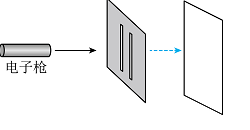$1.2×10^{-23} kg·m/s$，然后让它们通过双缝打到屏上。已知电子质量取$9.1×10^{-31}$ kg，普朗克常量取$6.6×10^{-34}J·s$，下列说法正确的是$\text{A.}$ 发射电子的动能约为 $8.0 \times 10^{-15} \mathrm{~J}$ $\text{B.}$ 发射电子的物质波波长约为 $5.5 \times 10^{-11} \mathrm{~m}$ $\text{C.}$ 只有成对电子分别同时通过双缝才能发生干涉 $\text{D.}$ 如果电子是一个一个发射的, 仍能得到干涉图样

BD

#### 解析：

A. 根据动量的大小与动能的关系可知发射电子的动能约为
$$E_{\mathrm{k}}=\frac{P^2}{2 m}=\frac{\left(1.2 \times 10^{-23}\right)^2}{2 \times 9.1 \times 10^{-31}} \mathrm{~J} \approx 8.0 \times 10^{-17} \mathrm{~J}$$

B. 发射电子的物质波波长约为
$$\lambda=\frac{h}{P}=\frac{6.6 \times 10^{-34}}{1.2 \times 10^{-23}} \mathrm{~m} \approx 5.5 \times 10^{-11} \mathrm{~m}$$

$\mathrm{CD}$. 物质波也具有波粒二象性, 故电子的波动性是每个电子本身的性质, 则每个电子依次通过双缝都能发